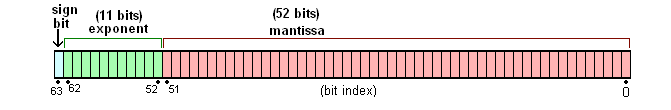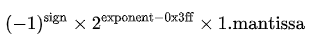# 前言

``0.1 + 0.2 = 0.30000000000000004;``

# IEEE 754 标准

IEEE 二进制浮点数算术标准（IEEE 754），是最广泛使用的浮点数运算标准，为许多 CPU 与浮点运算器所采用。

# 64 位的双精度• sign bit（S 符号）：符号位，表示正负号（0 为负数，1 为正数）
• exponent（E 指数）：表示次方数，在（二进制的）科学计数法中定义 2 的多少次幂
• mantissa（M 尾数）：表示精确度（小数部分，规范中会省略个位数上的 1 ）## 符号位 sign

``````Math.pow(-1, 0); //1
Math.pow(-1, 1); //-1``````

## 尾数位 mantissa

``````1000.001(2)
2^3 * 1.000001(科学计数法表示)``````

## 指数位 exponent

### 科学计数法

``````// 科学记数法
100; // 1*10^2
0.001; // 1*10^-3``````

``100(2); // 1*2^2``

### 指数偏移值（exponent bias）

``````# 补充下：为何负数是从 1024 开始？
011 11111111 # 1023

# 如果该二进制再继续增大，就进入了负数区域（最高位为 1）
100 00000000 # 1024``````

### 举例

``````4.5 (10)
100.1 (2)
1.001 * 2^2

# 解惑些问题

## 整数范围

``````(Math.pow(2, 53) - 1 == Number.MAX_SAFE_INTEGER; //9007199254740992
- (Math.pow(2, 53) - 1)) == Number.MIN_SAFE_INTEGER;//-9007199254740992``````

## 最小精度

``Math.pow(2, -52) == Number.EPSILON; //2.220446049250313e-16``

## 0.1+0.2 等于什么？

``````0.0001100110011001100110011001100110011001100110011001101 + 0.0011001100110011001100110011001100110011001100110011010 = 0.0100110011001100110011001100110011001100110011001100111;